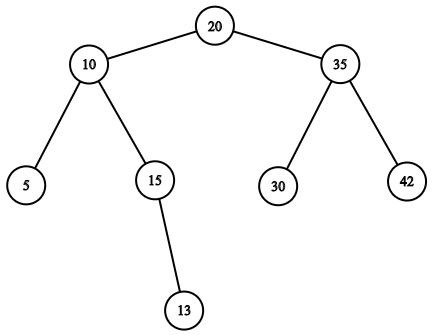# BST Delete

Posted: 7 Dec, 2020
Difficulty: Easy

## PROBLEM STATEMENT

#### A binary search tree (BST), also called an ordered or sorted binary tree, is a rooted binary tree whose internal nodes each store a key greater than all the keys in the node's left subtree and less than those in its right subtree.

##### Note:
``````All the elements of the binary search tree are unique.
``````
##### Input Format:
``````The first line of input contains an integer 'T', the number of test cases.

The first line of every test case contains elements in the level order form. The input consists of values of nodes separated by a single space in a single line. In case a node is null, we take -1 on its place.

The second line of every test case contains a single integer 'K' denoting the value of the node to be deleted. It is guaranteed that this value exists in the Binary Tree.

For example, the input for the tree depicted in the below image would be :
````````````20 10 35 5 15 30 42 -1 -1 -1 13 -1 -1 -1 -1 -1 -1
``````

#### Explanation :

``````Level 1 :
The root node of the tree is 20

Level 2 :
Left child of 20 = 10
Right child of 20 = 35

Level 3 :
Left child of 10 = 5
Right child of 10 = 15
Left child of 35 = 30
Right child of 35 = 42

Level 4 :
Left child of 5 = null (-1)
Right child of 5 = null (-1)
Left child of 15 = null (-1)
Right child of 15 = 13
Left child of 30 = null (-1)
Right child of 30 = null (-1)
Left child of 42 = null (-1)
Right child of 42 = null (-1)

Level 5 :
Left child of 13 = null (-1)
Right child of 13 = null (-1)

The first not-null node (of the previous level) is treated as the parent of the first two nodes of the current level.

The second not-null node (of the previous level) is treated as the parent node for the next two nodes of the current level and so on.

The input ends when all nodes at the last level are null (-1).
``````

#### Note :

``````The above format was just to provide clarity on how the input is formed for a given tree.

The sequence will be put together in a single line separated by a single space. Hence, for the above-depicted tree, the input will be given as:
20 10 35 5 15 30 42 -1 -1 -1 13 -1 -1 -1 -1 -1 -1
``````
##### Output Format:
``````For each test case, you need to return the head of the BST after deletion.
``````
##### Note:
``````You are not required to print the output explicitly, it has already been taken care of. Just implement the function.
``````
##### Constraints:
``````1 <= T <= 100
1 <= N <= 1000
1 <= data <= 10^9 and data != -1
1 <= K<= 10^9

Time limit: 1 second
``````Approach 1
• There can be three cases when a node is to be deleted:
• The node has 0 children/ node is a leaf. In this case, simply remove the node from the tree.
• The node has 1 child. In this case, delete the node and copy the child to this node.
• The node has 2 children. In this case, delete the node and copy the inorder successor to this node. (You could have also used the inorder predecessor).
• To find the inorder successor node, we take the leftmost node of the right child.
• We do this every time we find the node has two children.
• Finally delete the given node and return the head of the new Binary Tree.